# Graph, connectivity of a

(diff) ← Older revision | Latest revision (diff) | Newer revision → (diff)
One of the topological characteristics of a graph. A graph is said to be connected if for any two of its verticesandthere exists a chain connecting these vertices. The vertex connectivity number of a graph(denoted by) is the smallest number of vertices the removal of which results in a disconnected graph or in a graph consisting of one isolated vertex. The edge connectivity number (denoted by) is the smallest number of edges ofthe removal of which results in a disconnected graph. A graphis said to be-connected if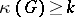, and-edge-connected if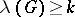. A maximal-connected subgraph of a graphis said to be a-connected component of it; a-connected component is called a connected component. In studying communication networks and logical networks, the connectivity numbers of the corresponding graphs can be interpreted as a degree of reliability of these networks.
The theory of graphs studies methods for determining the connectivity of graphs, conditions under which a graph is-connected or-edge-connected, the relations between the different types of connectivity, the dependence of the connectivity numbers on other graph parameters, etc. Thus, ifis the minimal degree of the vertices of a graph, the following inequalities are valid: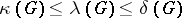.
For any integers(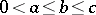) there exists a graphfor which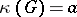,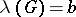,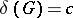. Ifhasvertices and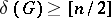, then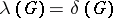. One says that a setof vertices, edges, or vertices and edges separates two verticesandifandbelong to different connected components of the graph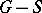obtained fromby the removal of the elements of. The following statements are valid.
The smallest number of vertices separating two non-adjacent verticesandis equal to the largest number of simple chains without common vertices connectingand. A graphis-connected if and only if any pair of its vertices is connected by at leastchains which do not intersect at the vertices. Similar theorems are also valid for edge connectivity. A graph is-edge-connected if and only if any pair of its vertices is connected by at leastchains which do not intersect at the edges. A set of edges whose removal results in the formation of a disconnected graph is known as a cut. In each graph the largest number of cuts which do not intersect at the edges and which separate two verticesandis equal to the smallest number of edges of a simple chain connectingand, i.e. to the distance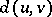betweenand.